SSC CGL Previous Year Questions: Ratio, Proportion, Mixture and Partnership - 3

# SSC CGL Previous Year Questions: Ratio, Proportion, Mixture and Partnership - 3 - SSC CGL

Test Description

## 31 Questions MCQ Test SSC CGL (Tier - 1) - Previous Year Papers (Topic Wise) - SSC CGL Previous Year Questions: Ratio, Proportion, Mixture and Partnership - 3

SSC CGL Previous Year Questions: Ratio, Proportion, Mixture and Partnership - 3 for SSC CGL 2023 is part of SSC CGL (Tier - 1) - Previous Year Papers (Topic Wise) preparation. The SSC CGL Previous Year Questions: Ratio, Proportion, Mixture and Partnership - 3 questions and answers have been prepared according to the SSC CGL exam syllabus.The SSC CGL Previous Year Questions: Ratio, Proportion, Mixture and Partnership - 3 MCQs are made for SSC CGL 2023 Exam. Find important definitions, questions, notes, meanings, examples, exercises, MCQs and online tests for SSC CGL Previous Year Questions: Ratio, Proportion, Mixture and Partnership - 3 below.
Solutions of SSC CGL Previous Year Questions: Ratio, Proportion, Mixture and Partnership - 3 questions in English are available as part of our SSC CGL (Tier - 1) - Previous Year Papers (Topic Wise) for SSC CGL & SSC CGL Previous Year Questions: Ratio, Proportion, Mixture and Partnership - 3 solutions in Hindi for SSC CGL (Tier - 1) - Previous Year Papers (Topic Wise) course. Download more important topics, notes, lectures and mock test series for SSC CGL Exam by signing up for free. Attempt SSC CGL Previous Year Questions: Ratio, Proportion, Mixture and Partnership - 3 | 31 questions in 31 minutes | Mock test for SSC CGL preparation | Free important questions MCQ to study SSC CGL (Tier - 1) - Previous Year Papers (Topic Wise) for SSC CGL Exam | Download free PDF with solutions
 1 Crore+ students have signed up on EduRev. Have you?
SSC CGL Previous Year Questions: Ratio, Proportion, Mixture and Partnership - 3 - Question 1

### 465 coins consists of 1 rupee, 50 paise and 25 paise coins. Their values are in the ratio 5 : 3 : 1. The number of each type of coins respectively is  (SSC CGL 2nd Sit.  2012)

Detailed Solution for SSC CGL Previous Year Questions: Ratio, Proportion, Mixture and Partnership - 3 - Question 1

The ratio of number of coins = 5 : 6 : 4
∴ The number of one rupee coins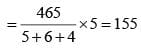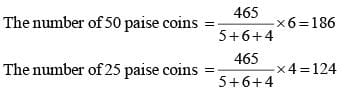SSC CGL Previous Year Questions: Ratio, Proportion, Mixture and Partnership - 3 - Question 2

### A, B, C subscribe together ` 50,000 for a business. A subscribes ₹ 4,000 more than B and B ₹ 5,000 more than C. Out of a total profit of ₹ 35,000, A receives    (SSC CGL 2nd Sit.  2012)

Detailed Solution for SSC CGL Previous Year Questions: Ratio, Proportion, Mixture and Partnership - 3 - Question 2

A = B + 4000 B = C + 5000
A + B + C = 50000
∴ A + A – 4000 + A – 9000 = 50000
So,  A = 21000
B = 17000
C = 12000
∴ A : B : C = 21000 : 17000 : 12000 = 21 : 17 : 12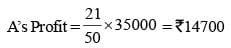SSC CGL Previous Year Questions: Ratio, Proportion, Mixture and Partnership - 3 - Question 3

### An employer reduces the number of employees in th e ratio 8 : 5 and increases their wages in the ratio 7 : 9. As a result, the overall wages bill is    (SSC CGL 1st Sit.  2012)

Detailed Solution for SSC CGL Previous Year Questions: Ratio, Proportion, Mixture and Partnership - 3 - Question 3

Required ratio = 8 × 7 : 5 × 9 = 56 : 45

SSC CGL Previous Year Questions: Ratio, Proportion, Mixture and Partnership - 3 - Question 4

A and B are partners in a business. A contributes 1/4 of the capital for 15 months and B received 2/3 of the profit. Find for how long B’s money was used?   (SSC CGL 1st Sit. 2012)

Detailed Solution for SSC CGL Previous Year Questions: Ratio, Proportion, Mixture and Partnership - 3 - Question 4

A’s profit : B’s profit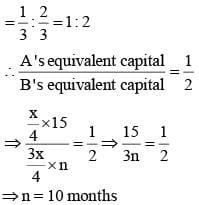SSC CGL Previous Year Questions: Ratio, Proportion, Mixture and Partnership - 3 - Question 5

A jar contains a mixture of two liquids A and B in the ratio 4 : 1. When 10 litre of the mixture is replaced with liquid B, the ratio becomes 2 : 3. The volume of liquid A present in the jar earlier was:    (SSC CGL 2nd Sit. 2012)

Detailed Solution for SSC CGL Previous Year Questions: Ratio, Proportion, Mixture and Partnership - 3 - Question 5

Liquid A = 4x litre
Liquid B = x litre
In 10 litres of  mixture,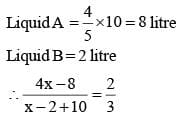⇒ 12x – 24 = 2x + 16
⇒ 10x = 40 ⇒ x = 4
∴ Initial quantity of liquid A = 16 litre

SSC CGL Previous Year Questions: Ratio, Proportion, Mixture and Partnership - 3 - Question 6

In a partnership business, A invests 1/6th of the capital for 1/6 of the total time, B invests 1/4 of the capital for 1/4 of the total time and C, the rest of the capital for the whole time. Out of a profit of  ₹ 19,400, B’s share is:     (SSC CGL 2nd Sit. 2012)

Detailed Solution for SSC CGL Previous Year Questions: Ratio, Proportion, Mixture and Partnership - 3 - Question 6

Ratio of profit sharing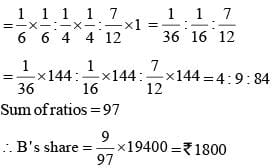SSC CGL Previous Year Questions: Ratio, Proportion, Mixture and Partnership - 3 - Question 7

The cost of a piece of diamond varies with the square of its weight. A diamond of ₹ 5,184 value is cut into 3 pieces whose weights are in the ratio 1 : 2 : 3. Find the loss involved in the cutting.    (SSC CGL 1st Sit. 2012)

Detailed Solution for SSC CGL Previous Year Questions: Ratio, Proportion, Mixture and Partnership - 3 - Question 7

If the weight of a piece of diamond be 6 x units, then
Original price k (6x)2 = 36kx2
∴ 36 kx2 = 5184 .....(i)
Again,
New price = k (x2 + 4x2 + 9x2) = 14 kx2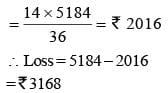SSC CGL Previous Year Questions: Ratio, Proportion, Mixture and Partnership - 3 - Question 8

The ratio in which a man must mix rice at ₹ 10.20 per kg and ₹ 14.40 per kg so as to make a mixture worth ₹ 12.60 per kg, is    (SSC Multitasking 2013)

Detailed Solution for SSC CGL Previous Year Questions: Ratio, Proportion, Mixture and Partnership - 3 - Question 8

By the rule of alligation: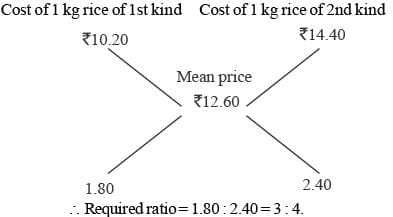SSC CGL Previous Year Questions: Ratio, Proportion, Mixture and Partnership - 3 - Question 9

A, B and C are batsmen. The ratio of the runs scored by them in a certain match are given below: A : B = 5 : 3 and B : C = 4 : 5. In all they scored 564 runs. The number of runs scored by B is:    (SSC CHSL 2012)

Detailed Solution for SSC CGL Previous Year Questions: Ratio, Proportion, Mixture and Partnership - 3 - Question 9

A : B = 5 : 3
B : C = 4 : 5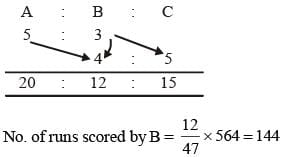SSC CGL Previous Year Questions: Ratio, Proportion, Mixture and Partnership - 3 - Question 10

In a school, the ratio of boys to girls is 4 : 3 and the ratio of girls to teachers is 8 : 1. The ratio of student to teachers is:    (SSC CHSL 2012)

Detailed Solution for SSC CGL Previous Year Questions: Ratio, Proportion, Mixture and Partnership - 3 - Question 10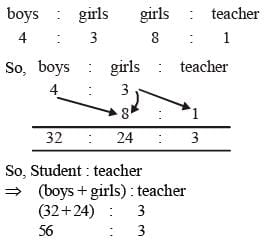SSC CGL Previous Year Questions: Ratio, Proportion, Mixture and Partnership - 3 - Question 11

A man leaves ₹ 12, 600 to be divided among 7 sons, 3 daughters and 5 nephews. If each daughter receives three times as much as each nephew and each son receives seven times as much as each nephew, then each daughter’s share is    (SSC Sub. Ins. 2012)

Detailed Solution for SSC CGL Previous Year Questions: Ratio, Proportion, Mixture and Partnership - 3 - Question 11

7S + 3D + 5N = ₹ 12600 ... (1)
According to Question,
D = 3N
S = 7N
Putting these values in equation (1)
49 N + 9N + 5N = 12600
⇒ 63N = 12600
⇒ N = 200
Each daughter’s share
= 3 × Nephew’s share = 3 × 200 = ₹ 600

SSC CGL Previous Year Questions: Ratio, Proportion, Mixture and Partnership - 3 - Question 12

A certain sum of money is distributed to A and B in the ratio 2 : 5. If A received ₹ 100, then the money received by B is    (SSC Sub. Ins. 2012)

Detailed Solution for SSC CGL Previous Year Questions: Ratio, Proportion, Mixture and Partnership - 3 - Question 12

Let ‘x’ be the total sum of money.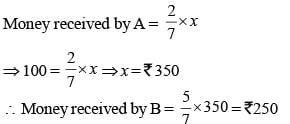SSC CGL Previous Year Questions: Ratio, Proportion, Mixture and Partnership - 3 - Question 13

Equal amounts of water were poured into two empty jars of different capacities, which made one jar 1/4 full and the other jar 1/3  full. If the water in the jar with lesser capacity is then poured into the jar with greater capacity, then the part of the larger jar filled with water is    (SSC Sub. Ins. 2012)

Detailed Solution for SSC CGL Previous Year Questions: Ratio, Proportion, Mixture and Partnership - 3 - Question 13

Amounts of water in two jars are equal; the jar with the greater capacity is 1/4 full, and the jar with lesser capacity is 1/3 full.
∴ When the water in smaller jar is poured into the larger jar, the addition of an equal amount of water will double the amount in the larger jar, which will then be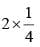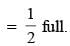SSC CGL Previous Year Questions: Ratio, Proportion, Mixture and Partnership - 3 - Question 14

Monthly incomes of A and B are in the ratio of 4 : 3 and their expenses bear the ratio 3 : 2. Each of them saves ₹ 6,000 at the end of the month, then the monthly income of A is    (SSC CGL 2nd Sit. 2011)

Detailed Solution for SSC CGL Previous Year Questions: Ratio, Proportion, Mixture and Partnership - 3 - Question 14

Let salary of A and B be 4x and 3x
expenditure = income-saving
ATQ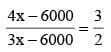⇒ 8x – 12000 = 9x – 18000
⇒ x = 6000
∴ A’s salary = 4x = 4 × 6000  = ₹ 24000

SSC CGL Previous Year Questions: Ratio, Proportion, Mixture and Partnership - 3 - Question 15

The ratio between two numbers is 2 : 3. If each number is increased by 4, the ratio between them becomes 5 : 7. The difference between the numbers is    (SSC CGL 2nd Sit. 2011)

Detailed Solution for SSC CGL Previous Year Questions: Ratio, Proportion, Mixture and Partnership - 3 - Question 15

Let the numbers be 2x and 3x.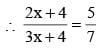∴ 15x + 20 = 14x +  28
⇒ x = 28 – 20 = 8 = Required Difference

SSC CGL Previous Year Questions: Ratio, Proportion, Mixture and Partnership - 3 - Question 16

The ratio of the quantities of an acid and water in a mixture is 1 : 3. If 5 litres of acid is further added to the mixture, the new ratio becomes 1 : 2. The quantity of new mixture in litres is    (SSC CGL 2nd Sit. 2011)

Detailed Solution for SSC CGL Previous Year Questions: Ratio, Proportion, Mixture and Partnership - 3 - Question 16

Let the quantity of acid in original mixture be x litre and that of water be 3x litre.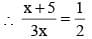⇒ 2x + 10 = 3x ⇒ x = 10
∴ Quantity of new mixture
= 4x + 5 = 45 litres

SSC CGL Previous Year Questions: Ratio, Proportion, Mixture and Partnership - 3 - Question 17

If x : y = 4 : 5, then  (3x + y) : (5x + 3y) =    (SSC CGL 2nd Sit. 2011)

Detailed Solution for SSC CGL Previous Year Questions: Ratio, Proportion, Mixture and Partnership - 3 - Question 17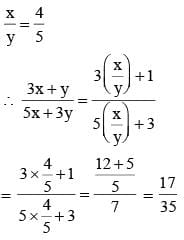SSC CGL Previous Year Questions: Ratio, Proportion, Mixture and Partnership - 3 - Question 18

Among three numbers, the first is twice the second and thrice the third. If the average of the three numbers is 49.5, then the difference between the first and the third number is     (SSC CGL 2nd Sit. 2011)

Detailed Solution for SSC CGL Previous Year Questions: Ratio, Proportion, Mixture and Partnership - 3 - Question 18

Let the second number be x.
∴ First number = 2x
∴ Third number = 2x/3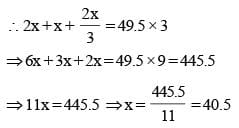∴ Requried difference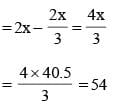SSC CGL Previous Year Questions: Ratio, Proportion, Mixture and Partnership - 3 - Question 19

The ratio of weekly incomes of A and B is 9 : 7 and the ratio of their expenditures is 4 : 3.  If each saves ₹ 200 per week, then the sum of their weekly incomes is    (SSC CGL 1st Sit. 2011)

Detailed Solution for SSC CGL Previous Year Questions: Ratio, Proportion, Mixture and Partnership - 3 - Question 19

Let monthly income of A and B be 9x and 7x
Expenditure = Income – Saving
ATQ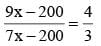⇒ 27x – 6.00 = 28x – 800
⇒ x = 200
∴ 200 × 16 =  ₹ 3200

SSC CGL Previous Year Questions: Ratio, Proportion, Mixture and Partnership - 3 - Question 20

What number should be added to or subtracted from each term of the ratio 17 : 24 so that it becomes equal to 1 : 2?    (SSC CGL 1st Sit. 2011)

Detailed Solution for SSC CGL Previous Year Questions: Ratio, Proportion, Mixture and Partnership - 3 - Question 20

Let the number x be added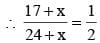⇒ 34 + 2x = 24 + x
⇒ 2x – x = 24 – 34
⇒ x = –10
Hence, 10 should be subtracted.

SSC CGL Previous Year Questions: Ratio, Proportion, Mixture and Partnership - 3 - Question 21

A can contains a mixture of two liquids A and B in the ratio 7 : 5. When 9 litres of mixture are drawn off and the can is filled with B, the ratio of A and B becomes 7 : 9. Litres of liquid A contained by the can initially was    (SSC CGL 1st Sit. 2011)

Detailed Solution for SSC CGL Previous Year Questions: Ratio, Proportion, Mixture and Partnership - 3 - Question 21

A = 7x litre, B = 5x litre (let)
In 9 litres of mixture,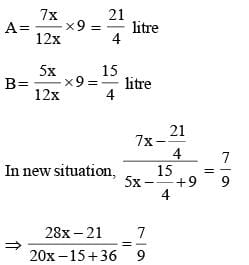SSC CGL Previous Year Questions: Ratio, Proportion, Mixture and Partnership - 3 - Question 22

In a business partnership among A, B, C and D, the profit is shared as follows: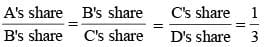If the total profit is ₹ 4,00,000, the share of C is    (SSC CGL 1st Sit. 2011)

Detailed Solution for SSC CGL Previous Year Questions: Ratio, Proportion, Mixture and Partnership - 3 - Question 22

A : B = 1 : 3
B : C = 1 : 3 = 3 : 9
C : D = 1 : 3 = 9 : 27
∴ A : B : C : D = 1 : 3 : 9 : 27
Sum of ratios = 1 + 3 + 9 + 27 = 40
∴ C’s share of profit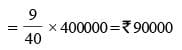SSC CGL Previous Year Questions: Ratio, Proportion, Mixture and Partnership - 3 - Question 23

If W1 : W2 = 2 : 3 and W1 : W3 = 1 : 2 then W2 : W3 is    (SSC CGL 2nd Sit. 2010)

Detailed Solution for SSC CGL Previous Year Questions: Ratio, Proportion, Mixture and Partnership - 3 - Question 23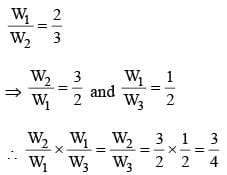SSC CGL Previous Year Questions: Ratio, Proportion, Mixture and Partnership - 3 - Question 24

The ratio of income and expenditure of a person is 11 : 10. If he saves ₹ 9,000 per annum, his monthly income is    (SSC CGL 2nd Sit. 2010)

Detailed Solution for SSC CGL Previous Year Questions: Ratio, Proportion, Mixture and Partnership - 3 - Question 24

Let the income of man be ₹ 11x and his expenditure be ₹ 10x.
∴ Savings x = ₹ 9000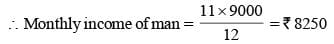Since income = 11x
expenditure = 10x
Saving = 11x – 10x = x
x = 9000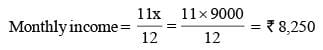SSC CGL Previous Year Questions: Ratio, Proportion, Mixture and Partnership - 3 - Question 25

Two numbers are in the ratio 1 : 3. If their sum is 240, then their difference is    (SSC CGL 2nd Sit. 2010)

Detailed Solution for SSC CGL Previous Year Questions: Ratio, Proportion, Mixture and Partnership - 3 - Question 25

Let the numbers be 3x and x.
3x + x = 240
⇒ 4x = 240
⇒ x = 240/4 = 60
∴ Difference = 3x – x = 2x = 2 × 60 = 120

SSC CGL Previous Year Questions: Ratio, Proportion, Mixture and Partnership - 3 - Question 26

The ratio of milk and water in mixtures of four containers are 5 : 3, 2 : 1, 3 : 2 and 7 : 4 respectively, in which container is the quantity of milk, relative to water, minimum?    (SSC CGL 2nd Sit. 2010)

Detailed Solution for SSC CGL Previous Year Questions: Ratio, Proportion, Mixture and Partnership - 3 - Question 26

Milk in V1 = 5/8 = 0.625
Milk in V2 = 2/3 = 0.66
Milk in V3 = 3/5 = 0.6
Milk in V4 = 7/11 = 0.636

SSC CGL Previous Year Questions: Ratio, Proportion, Mixture and Partnership - 3 - Question 27

If 1.5a = 0.04 b, then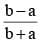is equal to   (SSC CGL 2nd Sit. 2010)

Detailed Solution for SSC CGL Previous Year Questions: Ratio, Proportion, Mixture and Partnership - 3 - Question 27

1.5a = 0.04 b
b/a = 1.5/ 0.04
By componendo and dividendo,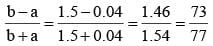SSC CGL Previous Year Questions: Ratio, Proportion, Mixture and Partnership - 3 - Question 28

Two number are in the ratio 7 : 11. If 7 is added to each of the numbers, the ratio becomes 2 : 3. The smaller number is    (SSC CGL 2nd Sit. 2010)

Detailed Solution for SSC CGL Previous Year Questions: Ratio, Proportion, Mixture and Partnership - 3 - Question 28

Let the numbers be 7x and 11x respectively.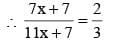∴ 22x + 14 = 21x + 21
⇒ x = 7
∴ Smaller number = 7x = 7 × 7 = 49

SSC CGL Previous Year Questions: Ratio, Proportion, Mixture and Partnership - 3 - Question 29

What must be added to each term of the ratio 7 : 11, so as to make it equal to 3 : 4?    (SSC CGL 1st Sit. 2010)

Detailed Solution for SSC CGL Previous Year Questions: Ratio, Proportion, Mixture and Partnership - 3 - Question 29

Let the required number be x.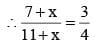⇒ 28 + 4x = 33 + 3x
⇒ x = 33 – 28 = 5

SSC CGL Previous Year Questions: Ratio, Proportion, Mixture and Partnership - 3 - Question 30

If  ₹ 1000 is divided between A and B in the ratio 3 : 2, then A will receive    (SSC CGL 1st Sit. 2010)

Detailed Solution for SSC CGL Previous Year Questions: Ratio, Proportion, Mixture and Partnership - 3 - Question 30

A’s share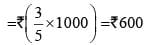SSC CGL Previous Year Questions: Ratio, Proportion, Mixture and Partnership - 3 - Question 31

A drum of kerosene is 3/4 full. When 30 litres of kerosene is drawn from it, it remains 7/12 full. The capacity of the drum is    (SSC CGL 1st Sit. 2010)

Detailed Solution for SSC CGL Previous Year Questions: Ratio, Proportion, Mixture and Partnership - 3 - Question 31

Let the capacity of the drum be x litres.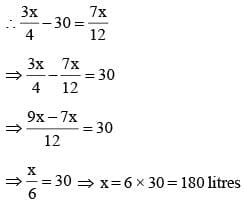## SSC CGL (Tier - 1) - Previous Year Papers (Topic Wise)

250 tests
Information about SSC CGL Previous Year Questions: Ratio, Proportion, Mixture and Partnership - 3 Page
In this test you can find the Exam questions for SSC CGL Previous Year Questions: Ratio, Proportion, Mixture and Partnership - 3 solved & explained in the simplest way possible. Besides giving Questions and answers for SSC CGL Previous Year Questions: Ratio, Proportion, Mixture and Partnership - 3, EduRev gives you an ample number of Online tests for practice

250 tests